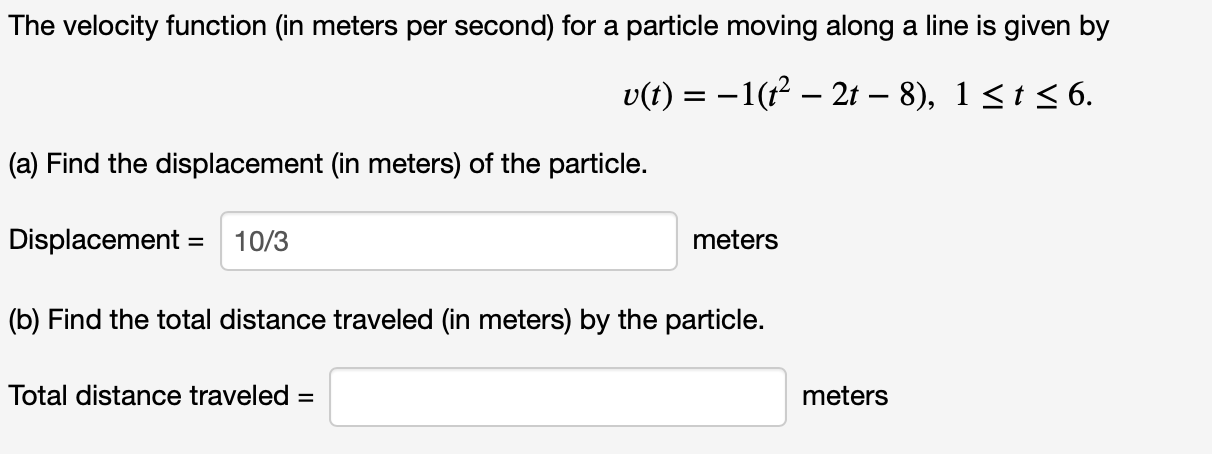# The velocity function (in meters per second) for a particle moving along a line is given by v(t)-1(2- 2t - 8), 1 st 6. (a) Find the displacement (in meters) of the particle Displacement 10/3 meters (b) Find the total distance traveled (in meters) by the particle. Total distance traveled = meters

Questionhelp_outlineImage TranscriptioncloseThe velocity function (in meters per second) for a particle moving along a line is given by v(t)-1(2- 2t - 8), 1 st 6. (a) Find the displacement (in meters) of the particle Displacement 10/3 meters (b) Find the total distance traveled (in meters) by the particle. Total distance traveled = meters fullscreen

### Want to see the step-by-step answer?